# Category Archives: Models

## A Math-History Timeline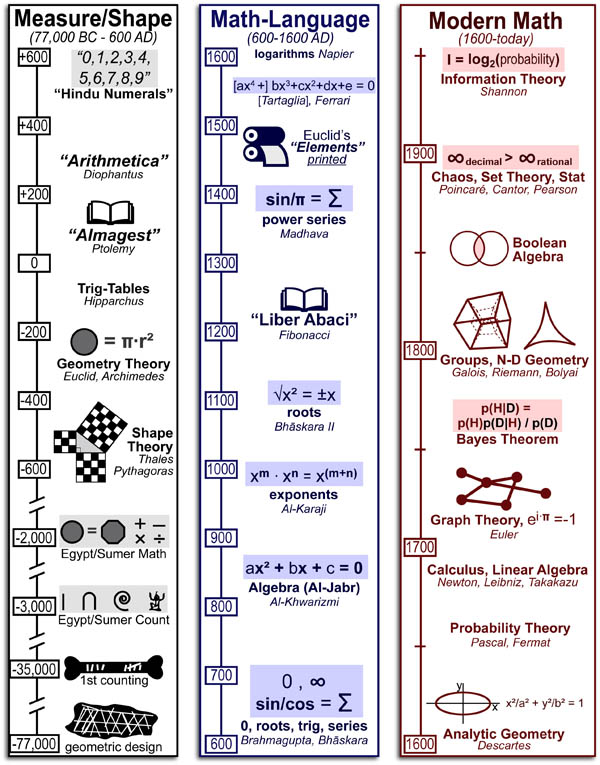The history of mathematics can be divided into three periods:

1. The Measurement and Shapes period (<77,000BC – 600AD): Mathematics first rose to preeminence with the agricultural revolution, around ~8,000BC, as a practical tool to organize economics and civilization(trade, accounting, taxes, etc.). Only with the Greeks (~600BC) did mathematics become a pure subject which was pursued for the purpose of “understanding”. Unfortunately, the Roman Empire did not share the Greek’s interest in “pure knowledge” and Europe forgot much what it had learned for the next 1000 years.

## Kinetics #6: Pulse-Chase Experiments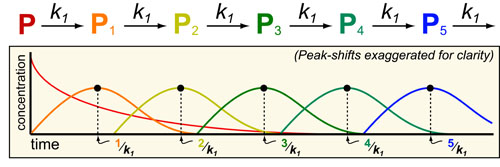In our sixth post on Understanding Kinetics, we consider pulse-chase experiments which are a common method to study bio-synthetic pathways. In pulse-chase experiments:

1. A “pulse” of labeled metabolite (P) is added to culture media.
2. The down-stream intermediates ((P1, P2, P3, etc.)) are measured (“chase“) with mass-spectrometry or radiation.

## Kinetics #5: Molecular Complexes (e.g. drug-target)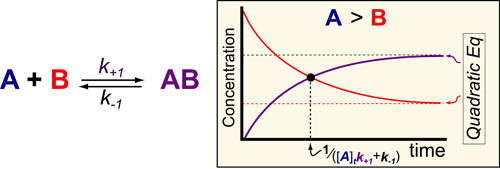In our fifth post on Understanding Kinetics, we consider the speed at which molecular complexes form. This is the fundamental mechanism underlying drug action (i.e. drugs inhibition their targets) and cellular signalling (i.e. ligands activate their receptors) and is probably the most important “kinetic effect” to consider in experimental design. Here again we use previously derived mathematical models1 to define some simple rules for the timescales/half-lives and magnitude of these reactions (figure above).

## Kinetics #4: Reversible On-Off States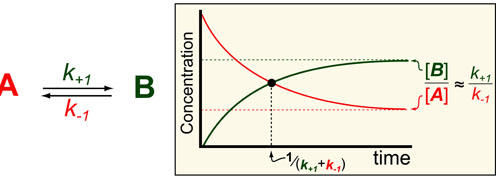In our fourth post in the Understanding Kinetics series we consider the speed at which proteins can turn off (A) or on (B). The dynamics of such processes are important to consider when designing experiments (i.e. How long should I wait to take a measurement?) and understanding Network Motifs in signalling cascades. Luckily we can use exact mathematical models (equations below1) for such processes to define some intuitive rules for the timescales/half-lives and magnitude of these reactions (figure above).

## What is Entropy??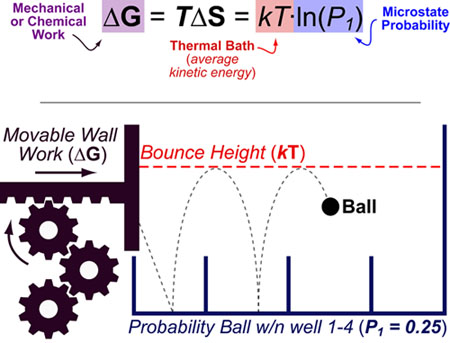Entropy (S) can be best understood as “the effect of probability on a physical or chemical processes”. This relationship is famously described by the Boltzmann entropy formula which relates the probability of a particular state (P1) to the chemical or mechanical work (ΔG) required to obtain that state.
Entropy changes(ΔS), are not probabilities per se but rather a conceptual bridge between probability and energy. In this equation, k is the Boltzmann constant, T is temperature, P is the probability of the considered state, ΔS is the entropy change and ΔG is the free energy change.

## The Maximal “Rate” of Evolution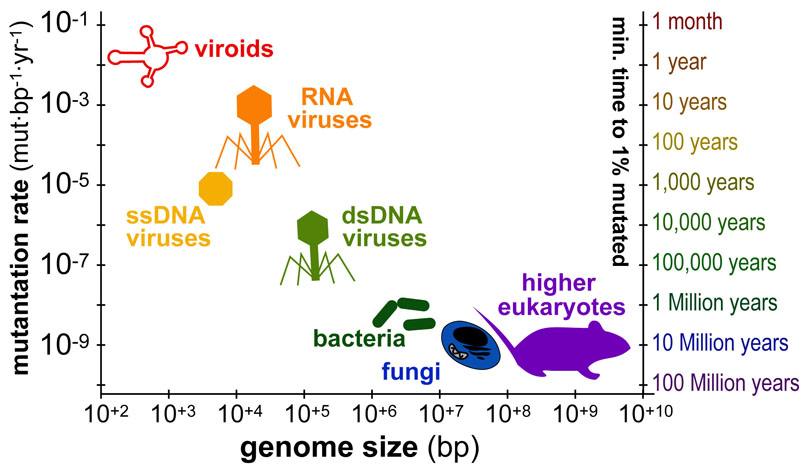The timescales over which organisms “mutate” or “evolve” varies dramatically from months(viruses) to millions of years(animals). The figure above plots the average genome size in base-pairs (x-axis) versus the average mutation-rate(y-axis) for various organisms. In addition, a second y-axis (“minimum time to 1% mutation”) illustrate approximate timescales corresponding to each rate.

## A Complete Aerobic Model of Marathon Performance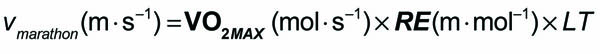The equation above is one of the simplest and most accurate predictors of marathon performance (having been shown to account for ~70% of the variability in individual marathon performance).1 In the figures below I delve more deeply into each of these terms to gain a better understanding of the molecular determinants of racing performance.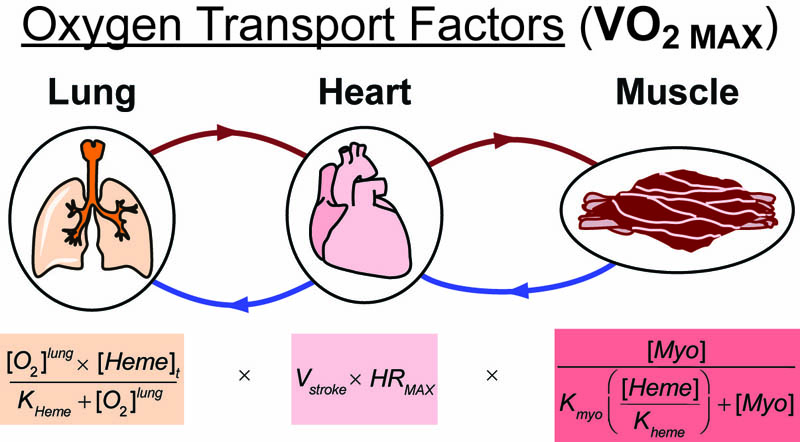## Understanding Reactivity with Hard-Soft Acid-Base Theory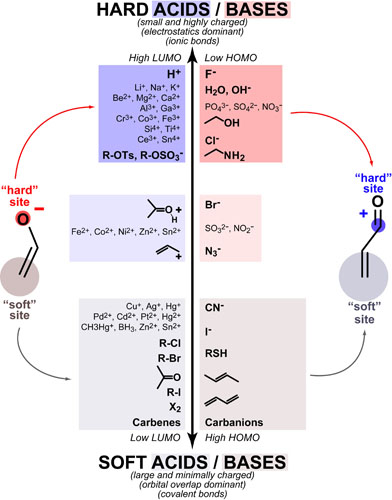Hard-Soft Acid-Base(HSAB) theory one of the most useful rules of thumb for explaining and predicting chemical reactivity trends. Hard molecules tend to be small/non-polarizable and charged while soft molecules tend to be large/polarizable and uncharged. Both acids/electrophiles and bases/nucleophiles can be hard and soft and the defining reactivity rule of HSAB theory is:

## Understanding Aromaticity based on Molecular Orbital Theory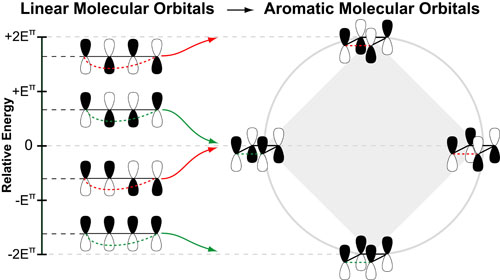Interestingly, once you understand the relative energies of linear pi-molecular orbitals the concept of “aromaticity” becomes alot simpler to understand. For example, cyclizing the frontier molecular orbitals (FMO) of butadiene gives you the anti-aromatic orbitals of cyclobutadiene. The “geometric arrangment” of these aromatic orbitals is a result of alternating stabilization (in green) or destabilization (in red) due to symmetry match or mismatch, respectively.1

## The Energies of Linear Frontier Molecular Orbitals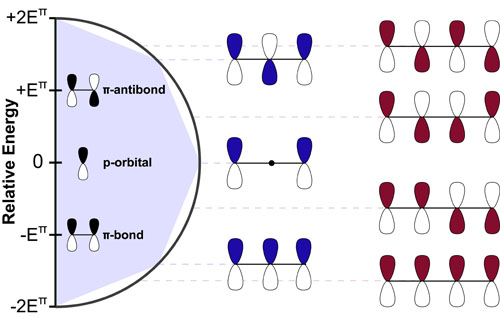The Woodward Hoffman rules are some of the most useful rules in organic chemistry. Unfortunately, because these rules are symmetry-based, they mostly ignore the relative energies of the molecular orbitals they consider. Luckily, Huckel theory (on which the Wood-ward Hoffman rules were based) gives as simple, geometric handle on the energies of these orbitals. Understanding these energies is critical for (1) rationalizing non-pericyclic reactivity trends and (2) answering the question: What exactly is aromaticity?

## Estimating Metabolite Concentrations at Steady StateIn a follow up to our post on sequential biochemical pathways, we next wanted to present an method to approximate the concentration of a metabolic intermediate in a biosynthetic pathway. In general, under steady state conditions, the steady state concentration of a metabolite can be estimated from the ratio of the Vmax for the upstream rate-determining enzyme over the rate of decay of that metabolite. A more complete equation is detailed below and further discussed in our post on Estimating Protein/Metabolite Levels from RNAseq data.

## Kinetics #3: Branch Points in Biochemical Pathways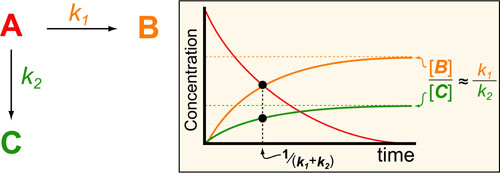In a follow up to our posts on Intuiting Enzyme Kinetics and Sequential Biochemical Pathways, we next wanted to consider the kinetic curves branch points in biochemical pathways (or equivalently kinetic competitions). Luckily exact mathematical models exist for competitive first-order processes (see below) and we can use these to develop intuitive rules (figure above) if we consider these pathways to be approximately pseudo-first order (which is often true in the context of biosynthetic pathways).

## Kinetics #2: Sequential Biochemical Pathways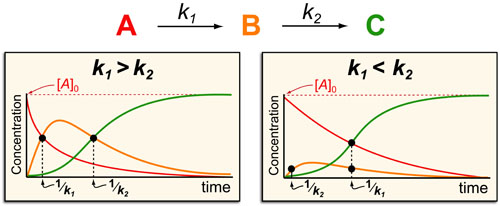In a follow up to our post our post on intuiting enzyme kinetics, we next wanted to consider the kinetic curves for sequential biochemical pathways. Luckily exact mathematical models exist for sequential first-order processes (see below) and we can use these to develop intuitive rules (figure above) if we consider these pathways to be approximately pseudo-first order (which is often true in the context of biosynthetic pathways).

## Kinetics #1: Catalyzed Reaction Timescales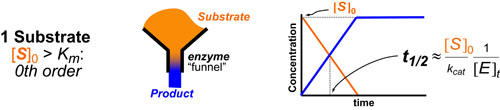Most chemical and biochemical research concerns reaction that are catalyzed by either an enzyme or a chemical catalyst. Unfortunately, when we learn chemical kinetics, it is usually in the context of idealized: uncatalyzed 0th-order, 1st-order of 2nd-order chemical reactions. Luckily the intuition we learn from 0 and 1st order processes can be readily extended to catalyzed processes by invoking the pseuso-1st order approximation. Below we describe how to “think about” the most common enzymatic/catalytic kinetic curves you may encounter.

## Understanding Multivalency (aka Avidity)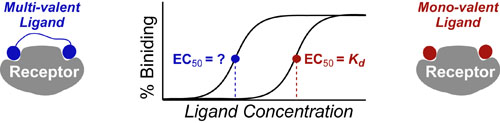Making a Ligand or Drug multivalent is a common method to try to improve the potency or EC50 of that drug from that predicted by the Hill Equation. Below we summarize the full spectrum of multivalent enhancement for the n = 2 case (n being the degree of multivalency) but these rules are easily extendable to the n-valent case aswell.